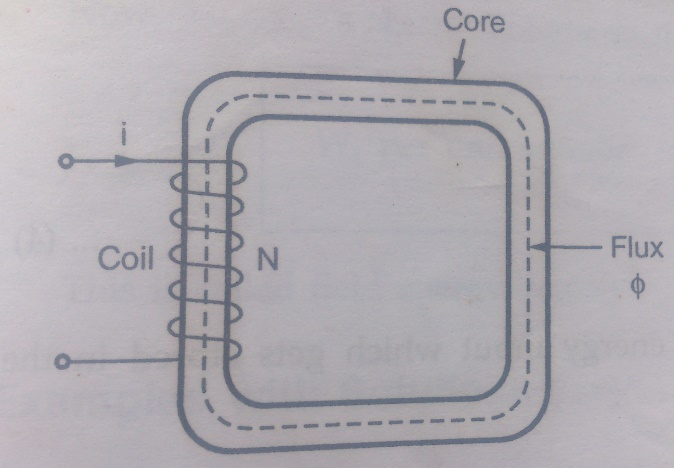Home | | Electrical Machines I | AC Operation of Magnetic Circuits

# AC Operation of Magnetic Circuits

For establishing a magnetic field, energy must be spent, though to energy is required to maintain it. Take the example of the exciting coils of an electromagnet.

AC Operation Of Magnetic Circuits

For establishing a magnetic field, energy must be spent, though to energy is required to maintain it. Take the example of the exciting coils of an electromagnet. The energy supplied to it is spent in two ways, (i) Part of it goes to meet I2R loss and is lost once for all (ii) part of it goes to create flux and is stored in the magnetic field as potential energy, and is similar to the potential energy of a raised weight, when a mass M is raised through a height of H, the potential energy stored in it is mgh. Work is done in raising this mass, but once raised to a certain height. No further expenditure of energy is required to maintain it at that position. This mechanical potential energy can be recovered so can be electric energy stored in a magnetic field. When current through an inductive coil is gradually changed from Zero to a maximum, value then every change of it is opposed by the self-induced emf. Produced due to this change. Energy is needed to overcome this opposition. This energy is stored in the magnetic field of the coil and is, later on, recovered when those field collapse.

In many applications and machines such as transformer and a.c machines, the magnetic circuits are excited by a.c supply. In such an operation, Inductance plays vital role even in steady state operation though in d.c it acts as a short circuit. In such a case the flux is determined by the a.c voltage applied and the frequency, thus the exciting current has to adjust itself according to the flux so that every time B-H relationship is satisfied.Consider a coil having N turns wound on iron core as shown in fig

The coil carries an alternating current i varying sinusoidally. Thus the flux produced by the exciting current I is also sinusoidally varying with time.

ϕm = Maximum value of flux in core

ω = 2πf where f is frequency in Hz

According to Faraday’s law as flux changes with respect to coli, the e.m.f gets induced in the coil given by,The sign of e.m.f induced must be determined according to len’s law, opposing the changes in the flux. The current and flux are in phase as current produces flux instantaneously. Now induced e.m.f is cosine term and thus leads the flux and current by .this is called back e.m.f as it opposes the applied voltage. The resistance drops is very small and is neglecte3d in most of the electromagnetic devices

## Energy stored under AC Operation

The instantaneous electric power input into the magnetic circuit through the coil terminals is given by,The power is rate of change of energy hence energy input which gets stored in the magnetic field during the interval t1 to t2 is,Thus Wf is the increase in the field energy as the flux linkages of the coil change from l1 to l2 during the interval t1 to t2 is,The flux density of the core is changing from B1 to B2 as the flux linkage change from  l1 to l2Thus is the field energy interms of field quantities.This is called fields energy density.

Study Material, Lecturing Notes, Assignment, Reference, Wiki description explanation, brief detail
Electrical machines : Magnetic Circuits and Magnetic Materials : AC Operation of Magnetic Circuits |